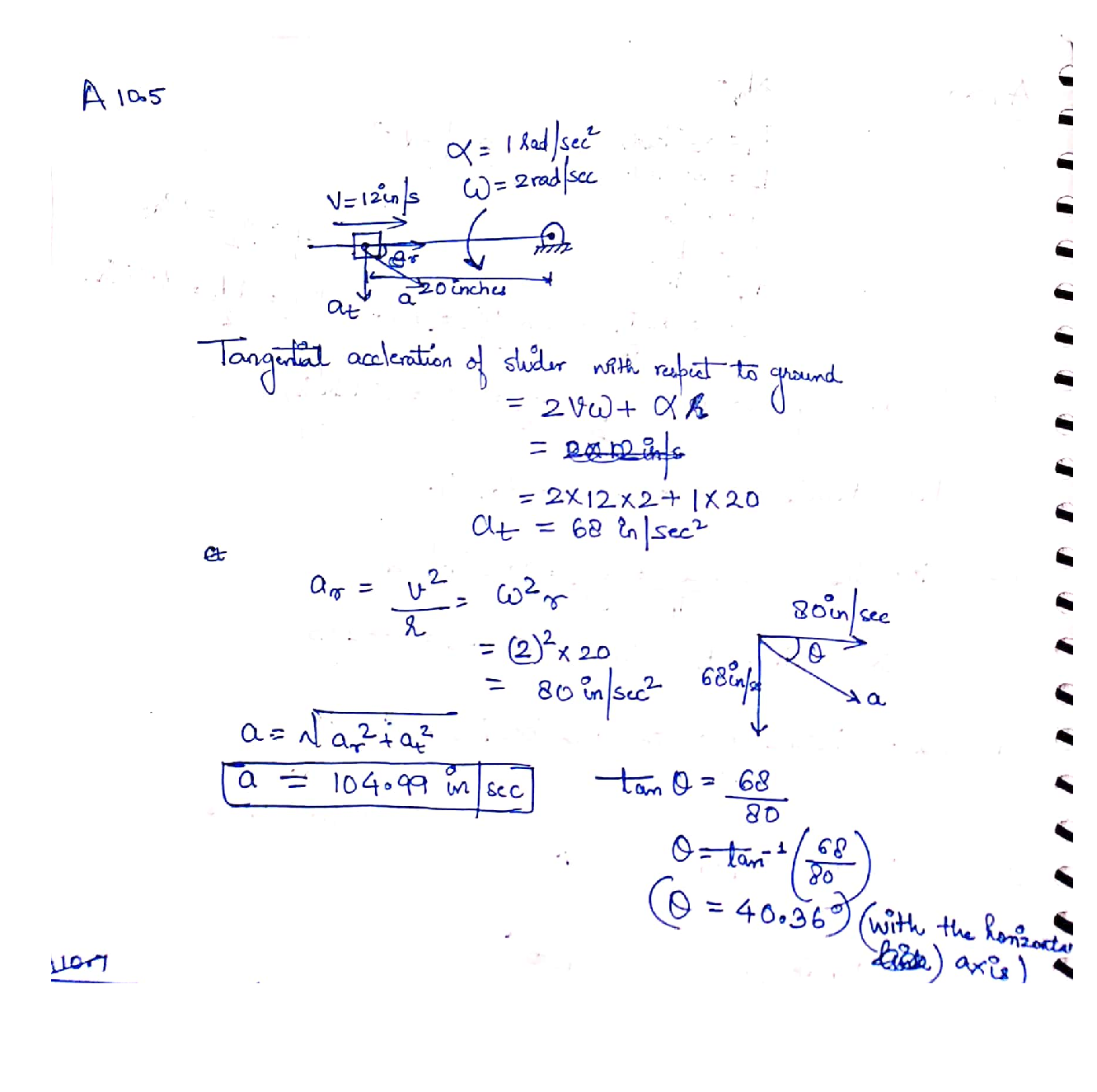Question

Kinematics of Machines

A10.5 - A 20-inch-long horizontal link is pinned to the ground on its right-hand end and rotates counterclockwise at 2 rad/s, accelerating 1 rad/s/s. A sliding link is attached to the other end of the horizontal link, traveling along it at a constant 12 in/s, toward the pinned end. Calculate the magnitude and direction of the acceleration of the slider with respect to the ground.Verified### Question 44189Kinematics of Machines

For the Planetary (Epicyclic) Gear, use Martin Table and calculate the velocity of each link, given the angular velocity of the input.

### Question 44188Kinematics of Machines

a. Determine the direction of the rotation by drawingan arrow on the gear.
b. Given the angular velocity of the input gear calculate the angular velocity of each link.
For the following gear set,

### Question 44187Kinematics of Machines

For the complex internal and external contact spur gear set,
a. Determine the direction of the rotation for each gear by drawing an arrow on it.
b. Calculate the transmission ratio between the input and the output gears as a function of Ni.
c. Determine the idler gear(s).

### Question 44186Kinematics of Machines

For each case,
a. Calculate the total number of instantaneous centers of rotation.
b. Specify the primary instantaneous centers of rotation and draw them on the circle diagram with solid lines.
c. Use Kennedy's theorem to find ONLY the secondary instantaneous center of rotation specified in the picture. Do this in the shortest possible way!

### Question 44185Kinematics of Machines

A. Calculate the total number of instantaneous centers of rotation.
B. Specify the primary instantaneous centers of rotation and draw them on the circle diagram with solid lines.
C. Use Kennedy's theorem to find all the secondary instantaneous centers of rotation and draw them on the circle diagram with dash lines.

### Question 44184Kinematics of Machines

Given the harmonic motion program, draw the corresponding cam contour.

### Question 44183Kinematics of Machines

\text { Given constant angular velocity }(\omega=1 \mathrm{rad} / \mathrm{sec}) \text { for the cam, }
Use two second order polynomials to design the motion program.а.
b. Plot position, velocity, acceleration, and jerk.
c.Approximately, draw the corresponding cam contour.

### Question 44182Kinematics of Machines

Only for inversion one of this mechanism,
A. Use Grash of condition and the function of the shortest link in the left- and right-hand side loops to examine the rotate ability of links # 2 and 6.
B. Use toggle position method in the left- and right-hand side loops to examine the rotate ability of links # 2 and 6.
C. If any of the links # 2 or 6 is a rocker determine the range of the rotation.

### Question 44181Kinematics of Machines

Choose your favorable Six-bar Mechanism from the videos on D2L or something you found in abook or online and then,
а.Provide the name and application of the mechanism.
b. Draw a schematic of the mechanism and specifying the input and output links.
c. Draw all the inversions of the mechanism.

### Question 43144Kinematics of Machines

Calculate the power N [W] necessary to maintain a constant speed of a rigid body with thedimensions shown in the figure, completely immersed, moving in a liquid with a density ofp [kg/m³] and a kinematic viscosity coefficient v[m² /s] at the speed v [m/s]. For the calculations,take:
C_{x}=0,025
v=10^{-6}\left[\mathrm{~m}^{2} / \mathrm{s}\right]
v=10-0,2 \cdot N r[m / s]
L=2+N r[m]
\rho=1025\left[k g / m^{3}\right]
D=0,8+0,2 \cdot N r[m]

### Submit query